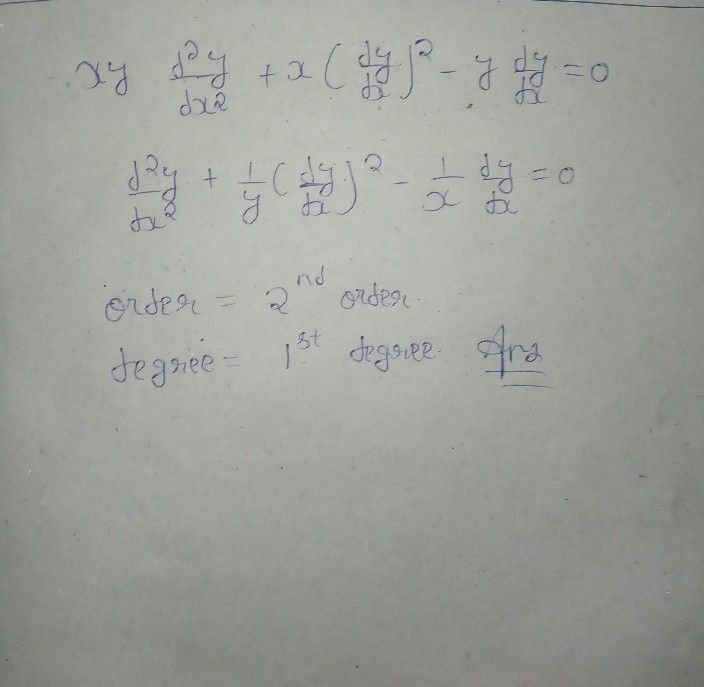Symbol
Problem$20$ Find the order and degree of the differential equaiton $xy\dfrac {d^{2}y} {dx^{2}}$ $+.x\left(\dfrac {dy} {dx}\right)^{2}$ $→y.\dfrac {dy} {dx}.=0$
10th-13th grade
Calculus
Search count: 106
SolutionQanda teacher - Trishaplz give 5 star rating